0
1480

# Top 50 Most Important SNAP Quant Questions

Download Top 105 Quant Questions for SNAP 2022. Very important and most expected Quantitavie aptitude Questions and answers based on asked questions in previous exam papers.

Question 1: We are told that f(x) is a polynomial function such that f(a)f(b) = f(a) + f(b) + f(ab) – 2 and f(4) = 17, find the value of f(7).

Solution:

f(a)f(b) = f(a) + f(b) + f(ab) – 2
Put a = b = 1.
$[f(1)]^2 = 3f(1) – 2$ => f(1) = 1 (or) 2.
Let’s assume f(1) = 1
Now, put b = 1.
f(a) = 2f(a) – 1
=> f(a) = 1 => For all values of a, f(a) = 1.
This is false because f(4) = 17.
=> f(1) = 2 is the correct value.
Now put b = 1/a
f(a)f(1/a) = f(a) + f(1/a) + 2 – 2
=> f(a)f(1/a) = f(a) + f(1/a)

So taking RHS terms to LHS and adding 1 to both sides we get
f(a)f(1/a) – f(a) – f(1/a) +1 = 1
(f(a) – 1) (f(1/a)-1) = 1
Let g(x) = f(x)-1
So g(x)*g(1/x) = 1
So g(x) is of the form $\pm x^n$
So f(x) is of the form $\pm x^n + 1$.

f(a) = $\pm a^n + 1$ satisfies the above condition.
$-4^n + 1$ = 17 => $-4^n$ = 16, which is not possible.
$4^a + 1$ = 17 => n = 2
=> f(a) = $a^2 + 1$
=> f(7) = $7^2 + 1$ = 50

Question 2: A takes 16 days more and B takes 4 days more than the number of days taken by both of them if they work together, to complete a piece of work. How many days does A take to complete the same piece of work, working alone?

Solution:

Suppose they take x days together to complete the work.
1/x = (1/(x+4))+ 1/(x+16)
x = 8 satisfies the given equation.
Time taken by A = 8+16 = 24 days.

Question 3: The mean score of ten batsmen of Hyderabad cricket team in a match is 60. If the top five scores of the batsmen are removed while calculating the average, the average falls by five. If the top six scores are distinct integers, what is the maximum possible score of any batsmen?

a) 102

b) 87

c) 95

d) 126

Solution:

Total score of the 10 batsmen = 600
Average of bottom five batsmen = 55
Total score of bottom five batsmen = 275
Total score of top 5 batsmen = 325
To obtain the maximum score, the scores of others are to be minimised
The minimum score of the top five batsmen can be 56 when all the bottom five batsman have 55 as their score.
Hence, 56,57,58,59,95 are the scores of the top five batsmen.

Question 4: A square ABCD in inscribed inside a circle. A circle is inscribed in the square ABCD. This process is repeated infinitely. What is the sum of areas of all the circles, if the radius of the first circle is 5cm?

a) $100\pi$

b) $25\pi$

c) $50\pi$

d) $45\pi$

Solution:

The diagonal of the first square is the diameter of the first circle = 10. The side of the first square is the diameter of the second circle = $10/\sqrt2$. The area of the first circle is $25\pi$. The area of the second circle = $25\pi$/2. Therefore, the sum of areas = $25\pi$ + $25\pi$/2 + $25\pi$/4 +…. = $50\pi$.

Question 5: The value of $1^3-2^3+3^3-4^3………………….-100^3$ is

a) -507500

b) -681750

c) -676700

d) -504000

Solution:

$1^3-2^3+3^3-4^3………………….-100^3$

=$1^3+2^3+3^3…….100^3-2\left(2^3+4^3+6^3………100^3\right)$

=$1^3+2^3+3^3…….100^3-2\times\ 2^3\left(1^3+2^3+3^3………50^3\right)$

=$\left(\ \frac{\ 100\times\ 101}{2}\right)^2\ -\ 16\left(\ \frac{\ 50\times\ 51}{2}\right)^2$

=$50^2\times\ 101^{2\ }-4\times\ 50^2\times\ 51^2$

=$50^2\left(101^{2\ }-2^2\times\ 51^2\right)$

= $50^2\left(101-102\right)\left(101+102\right)$

=-507500

Question 6: What is 7330th term in the sequence 2, 2, 4, 4, 4, 4, 6, 6, 6, 6, 6, 6, 8, . . . . . is?

Solution:

We see that 2 is repeated twice. So use of 2 ends in 2nd term.
Then 4 is repeated four times. So use of 4 ends in 2+4 i.e 6th term
Then 6 is repeated six times. So use of 6 ends in 2+4+6 i.e 12th term
And so on
.
.
Then 170 is repeated 170 times. So use of 170 ends in 2+4+6+…+170
Now, 2+4+6+…+170 = 7310
7311th term would be 172 and will be repeated 172 times.
So 7330th term must be 172.

Question 7: A dishonest milkman dilutes milk with water and then sells the diluted milk at 25% more than the price at which he purchased the milk. If he makes a profit of 40% by implementing this process. Find the volume of water(in ml) added to every litre of pure milk (Assume water is free of cost)

Solution:

Let the cost price of 1 litre of pure milk = Rs. 12 (value taken for easier calculations)

Then the selling price of 1 liter of solution= Rs 15

y be the volume of water added to every 1 litre of solution => SP of (1+y) litres of solution = 15 x (1+y)

$\frac{(1+y)15 – 12}{12}$ = 0.4

y = $\frac{1.8}{15}$

The volume of water added in ml = $\frac{1.8}{15}$*1000 = 120ml

Question 8: In a quadilateral PQRS, T is a point on the side PQ such that $\angle$PST = $\angle$STR = $\angle$TRQ = 45°. If PS = 16 cm and RQ = 24 cm then find out the area of triangle TRS?

a) $72\sqrt{2}$ sq. cm

b) $192\sqrt{2}$ sq. cm

c) $144\sqrt{2}$ sq. cm

d) $96\sqrt{2}$ sq. cm

Solution:

We are given that $\angle$PST = $\angle$STR ,therefore, we can say that PS $\parallel$ TR

Similarly, $\angle$STR = $\angle$TRQ, TS $\parallel$ QR

In triangles PST and TRQ,

TS $\parallel$ QR , PS $\parallel$ TR  and  $\angle$PST = $\angle$TRQ

Hence, we can say that $\triangle$PST $\sim$ $\triangle$TRQ.

Therefore, we can say that $\dfrac{PS}{ST} = \dfrac{TR}{RQ}$

$\Rightarrow$ ST*TR = PS*RQ  = 16*24 = 384 sq. cm

We know that area of triangle TRS = $\dfrac{1}{2}*ST*TR*\sin$45° = $\dfrac{1}{2\sqrt{2}}*ST*TR$ = $\dfrac{1}{2\sqrt{2}}*384$ = $96\sqrt{2}$ sq. cm

Thus, option D is the correct answer.

Question 9: Mohd. Ismail has a big family including one kid. After he lost his employment, he was dependant entirely on his bank balance to support his family. The balance he had would support his family for 90 days. After 40 days of losing his job, 4 guests visited his house and the balance was empty in 75 days from the day he lost his job. Assuming that the expenses were proportional to the number of family members and guests and that the kid uses exactly onethird of the expenses as the other members, what was the original number people in Ismail’s family (Count the kid as one member)?

Solution:

Let each member of the family apart from the kid use Rs. 3x in a day.
Let the kid of the family use Rs. x in a day.

Let there be ‘n’ members and a kid in the family initially.

Total bank balance = (n*3x+1*x) * 90 = 270nx+90x
Rupees used in 40 days = 40*n*3x + 40*1*x= 120nx+40x

The amount used by the guests and family members = 35*(n+4)*3x+35x = 105nx+455x

But (120nx+40x) + (105nx+455x) = 270nx+90x

45nx = 405x
n = 405/45 = 9

So, there are 10 members in the family including the kid.

Question 10: A solid right circular cone of height 27 cm is cut into two pieces along a plane parallel to its base at a height of 18 cm from the base. If the difference in volume of the two pieces is 225 cc, the volume, in cc, of the original cone is

a) 243

b) 232

c) 256

d) 264

Solution:

Let the base radius be 3r.

Height of upper cone is 9 so, by symmetry radius of upper cone will be r.

Volume of frustum=$\frac{\pi}{3}\left(9r^2\cdot27-r^2.9\right)$

Volume of upper cone = $\frac{\pi}{3}.r^2.9$

Difference= $\frac{\pi}{3}\cdot9\cdot r^2\cdot25=225$ => $\frac{\pi}{3}\cdot r^2=1$

Volume of larger cone = $\frac{\pi}{3}\cdot9r^2\cdot27=243$

Question 11: In a group of 10 students, the mean of the lowest 9 scores is 42 while the mean of the highest 9 scores is 47. For the entire group of 10 students, the maximum possible mean exceeds the minimum possible mean by

a) 5

b) 4

c) 3

d) 6

Solution:

Let x(1) be the least number and x(10) be the largest number. Now from the condition given in the question , we can say that

x(2)+x(3)+x(4)+……..x(10)= 47*9=423……………….(1)

Similarly x(1)+x(2)+x(3)+x(4)…………….+x(9)= 42*9=378……………(2)

Subtracting both the equations we get x(10)-x(1)=45

Now, the sum of the 10 observations from equation (1) is 423+x(1)

Now the minimum value of x(10) will be 47 and the minimum value of x(1) will be 2 . Hence minimum average 425/10=42.5

Maximum value of x(1) is 42. Hence maximum average will be 465/10=46.5

Hence difference in average will be 46.5-42.5=4 which is the correct answer

Question 12: The number of integral solutions of the equation $2^x(4-x) = 2(x+2)$ is

Solution:

$2^x$ > 0

So $\frac{2(x+2)}{4-x}$ > 0

$(x+2)(4-x)$ > 0

-2 < x < 4

From this range of values only x = 0, 1, 2 satisfies the equation.

Hence 3 is the correct answer.

Question 13: In triangle ABC, two points P and Q are on AB and BC respectively such that AP:BP=1:4 and BQ:CQ=2:3. The ratio of areas of triangle BPQ and the quadrilateral PQCA is

a) $\frac{8}{25}$

b) $\frac{17}{25}$

c) $\frac{9}{17}$

d) $\frac{8}{17}$

Solution: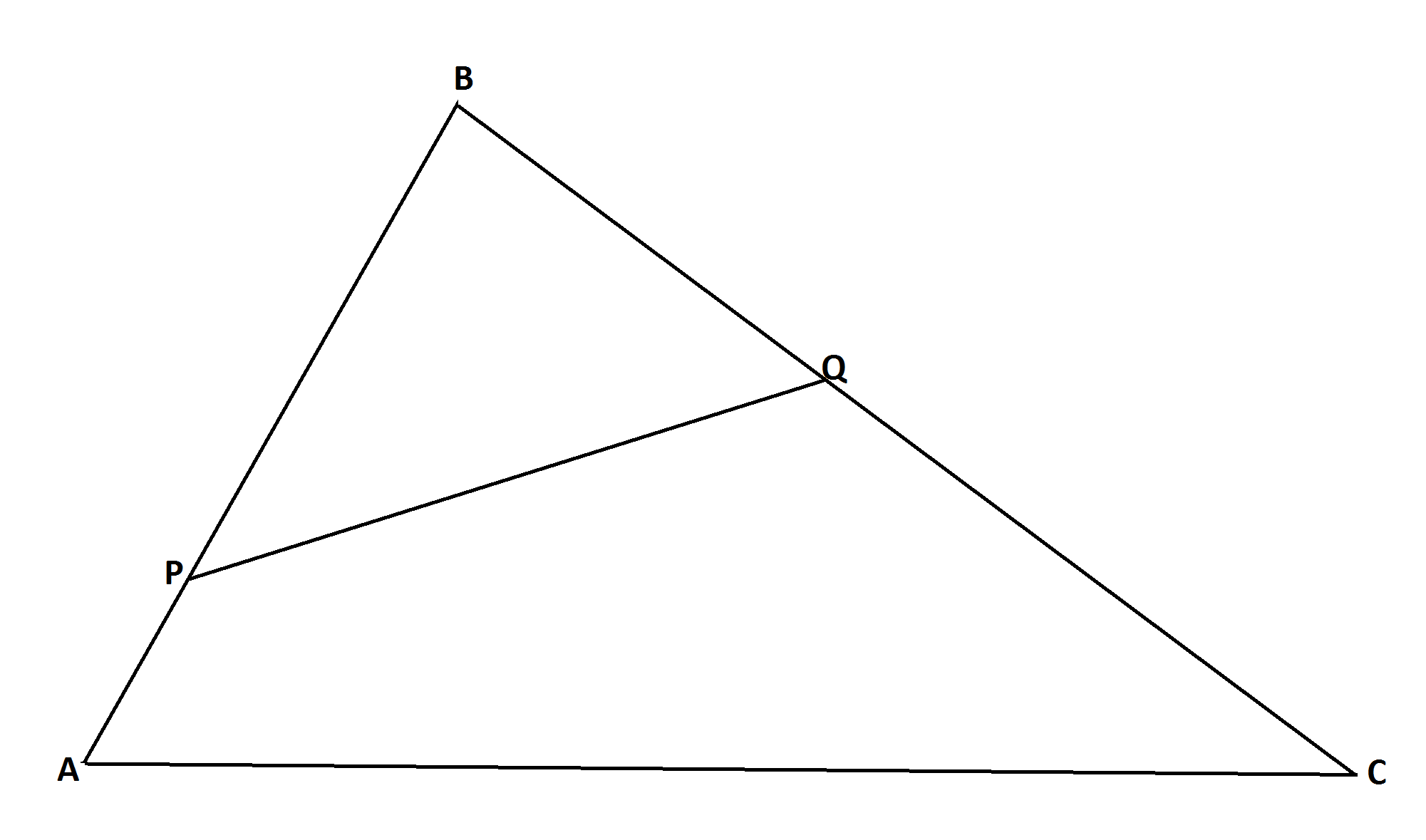We have AP:PB=1:4 and BQ:CQ=2:3

Now the area of ABC=$\frac{1}{2}BA*BCsin \theta$ = x (Assume)

Similarly, area of BPQ=$\frac{1}{2}BP*BQsin \theta$ = $\frac{1}{2}(\frac{4}{5}BA)*(\frac{2}{5}BC)sin \theta$ = $\frac{8}{25}x$

Now,$\frac{area BPQ}{area PQCA}$ = $\frac{area BPQ}{area ABC – area BPQ}$ = $\frac{\frac{8}{25}x}{x-\frac{8}{25}x}$ = $\frac{8}{17}$

Question 14: Aditya, Bikas and Chandu together take 12 days to complete a work. The time taken by Aditya and Bikas is 25% of the time taken by Chandu alone to complete the work. Time taken by Chandu and Bikas to complete the work is equal to the time taken by Aditya alone to complete the work. Find the time taken by Aditya and Chandu to complete the work when Aditya works at 80% of his actual efficiency while Chandu works at his actual efficiency.

a) 24

b) 15

c) 20

d) $\frac{120}{7}$

Solution:

Let us assume Aditya does a units of work per day, Bikas does b units of work per day and Chandu does c units of work per day.
Now total work would be: 12(a+b+c)
Now as per given condition :
we get,
$\frac{\left(a+b\right)}{4}=c$ and     (1)
b+c=a                                                   (2)
Putting a=b+c in (1)
we get
2b=3c
$\frac{b}{c}=\frac{3}{2}$
a:b:c=5:3:2
Now total time taken by Aditya and Chandu to complete the work if Aditya works at 80% of his efficiency and Chandu works at his actual efficiency would be:
$\frac{\left(12\left(5+3+2\right)\right)}{5\times\ 0.8+2}$
$\frac{120}{6}=20$ days.

Question 15: John takes twice as much time as Jack to finish a job. Jack and Jim together take one-thirds of the time to finish the job than John takes working alone. Moreover, in order to finish the job, John takes three days more than that taken by three of them working together. In how many days will Jim finish the job working alone?

Solution:

Let Jack take “t” days to complete the work, then John will take “2t” days to complete the work. So work done by Jack in one day is (1/t) and John is (1/2t) .

Now let Jim take “m” days to complete the work. According to question, $\frac{1}{t}+\frac{1}{m}=\frac{3}{2t}\ or\ \frac{1}{m}=\frac{1}{2t\ }or\ m=2t$ Hence Jim takes “2t” time to complete the work.

Now let the three of them complete the work in “p” days. Hence John takes “p+3” days to complete the work.

$\frac{1}{2t}\left(m+3\right)=\left(\frac{4}{2t}\right)m$

$\frac{1}{2t}\left(m+3\right)=\left(\frac{4}{2t}\right)m$

or m=1. Hence JIm will take (1+3)=4 days to complete the work. Similarly John will also take 4 days to complete the work

Question 16: An infinite geometric progression has the sum 10. Sum of all the possible integral values of the first term is

Solution:

Let us consider the first term of GP=a

Common ratio=r

$\frac{a}{1-r}$=10

r=1-$\frac{a}{10}$

-1 < r< 1

-1 < 1-$\frac{a}{10}$ < 1

0 < a < 20 but the value of a cannot be 10

Sum of possible integral values of a =Sum of first 19 Natural Numbers – 10

=$\frac{19*20}{2}$ – 10

=180

Question 17: A square of length 1 m is inside a square of length 2 m and four quarter circles are joined as shown in the figure. The value of y —x is given by,

a) $\frac{8-\pi}{10}$

b) $\frac{4-\pi}{5}$

c) $\frac{2\pi-1}{8}$

d) $\frac{\pi-3}{4}$

Solution:

From the above figure area of  the region bounded by ABCDEFGH = Are of the square with side 2 – (4*quadrants with side 1cm + 4x)

=4-$\pi\$-4x

Which is same as the area of the square with side 1 cm -4y

4-$\pi\$-4x = 1-4y

4x-4y=3-$\pi$

y-x= $\frac{\pi-3}{4}$

Question 18: The cost of 4 pens, 10 pencils and 14 erasers at a stationery shop is Rs 120. At the same shop, the cost of 6 pens and 10 erasers is Rs 60 more than 12 pencils. By how much amount (in Rs.) does the cost of 78 pencils and 2 erasers exceed the cost of 12 pens?

Solution:

Let the cost of a pen be x, the cost of a pencil be y and the cost of an eraser be z.
4x + 10y + 14z = 120
=> 2x + 5y + 7z = 60 → (1)
6x + 10z = 60 + 12y
=> 6x – 12y + 10z = 60
=> 3x – 6y + 5z = 30 → (2)
We need the value of 78y + 2z – 12x = 2*(-6x + 39y + z)
3*(1) – 4*(2) gives 6x + 15y + 21z – 12x + 24y – 20z = 180 – 120
=> -6x + 39y + z = 60
So, the required value is 2*60 = 120

Question 19: A contractor agreed to construct a 6 km road in 200 days. He employed 140 persons for the work. After 60 days, he realized that only 1.5 km road has been completed. How many additional people would he need to employ in order to finish the work exactly on time?

Solution:

Let the desired efficiency of each worker ‘6x’ per day.

140*6x*200= 6 km …(i)

In 60 days 60/200*6=1.8 km of work is to be done but actually 1.5km is only done.

Actual efficiency ‘y’= 1.5/1.8 *6x =5x.

Now, left over work = 4.5km which is to be done in 140 days with ‘n’ workers whose efficiency is ‘y’.

=> n*5x*140=4.5 …(ii)

(i)/(ii) gives,

$\frac{\left(140\cdot6x\cdot200\right)}{\left(n\cdot5x\cdot140\right)}=\frac{6}{4.5}$

=> n=180.

.’. Extra 180-140 =40 workers are needed.

Question 20: For a natural number X, P(X) represents the probability that a palindrome natural number (chosen randomly) less than X is divisible by 11. Then,

a) P(5000) > P(1000) > P (10000)

b) P(10000) > P(5000) > P(1000)

c) P(1000) > P(100) > P(10)

d) P(100)>P(10)>P(1000)

Solution:

An even digit palindrome number is always divisible by 11, while an odd digit palindrome number is not always divisible by 11.
So, P(10000) > P(5000) > P(1000).

Question 21: N is a multiple of 72. Also, it is known that all the digits of N are different. What is the largest possible value of N?

Solution:

We know that the number is a multiple of 72. Therefore, the number must be a multiple of both 8 and 9.
If the number contains all the digits from 0 to 9, the sum of the digits will be 9*10/2 = 45.
Therefore, a number containing all the ten digits will be divisible by 9.
For a number to be divisible by 8, the last 3 digits should be divisible by 8.
Now, we have to find the largest possible number. Therefore, the left-most digit must be 9, the second digit from the left should be 8 and so on.
We’ll get 9876543210 as the number. However, the last 3 digits are not divisible by 8. We must try to make the last 3 digits divisible by 8 without altering the position of other numbers. ‘120’ is divisible by 8.

Therefore, the largest number with different digits divisible by 72 is 9876543120.

Question 22: In a circle, two chords AB and CD intersect at a point E as shown in the figure. If AB = 6 cm and CD = 10 cm and $\angle$ OED = 30°, then find out the radius of the given circle.

a) $\sqrt{34}$ cm

b) $\sqrt{31}$ cm

c) $\sqrt{33}$ cm

d) $4\sqrt{2}$ cm

Solution:

Let us draw perpendicular from centre of the circle to AB and CD.

We are given that $\angle$ OED = 30°, therefore $\angle$ AOE =90°-30° = 60°.

Let ‘R’ be the radius of the given circle. Then, in right-angle triangle ODF,

$\Rightarrow$ $OF^2=OD^2-FD^2$

$\Rightarrow$ $OF^2=R^2-5^2$

$\Rightarrow$ $OF=\sqrt{R^2-25}$ cm.

In right-angle triangle OFE,

$\Rightarrow$ $sinOEF=\dfrac{OF}{OE}$

$\Rightarrow$ $\dfrac{1}{2}=\dfrac{OF}{OE}$

$\Rightarrow$ $OE=2*\sqrt{R^2-25}$  … (1)

Similarly, in right-angle triangle OGA,

$\Rightarrow$ $OG^2=OA^2-AG^2$

$\Rightarrow$ $OG=\sqrt{R^2-9}$

In right-angle triangle OGE,

$\Rightarrow$ $sinOEG=\dfrac{OG}{OE}$

$\Rightarrow$ $\dfrac{\sqrt{3}}{2}=\dfrac{OG}{OE}$

$\Rightarrow$ $OE=\dfrac{2}{\sqrt{3}}*\sqrt{R^2-9}$  … (2)

By equating (1) and (2)

$\Rightarrow$ $2*\sqrt{R^2-25}=\dfrac{2}{\sqrt{3}}*\sqrt{R^2-9}$

$\Rightarrow$ $3*(R^2-25)=R^2-9$

$\Rightarrow$ $2R^2=66$

$\Rightarrow$ $R=\sqrt{33}$ cm. Hence, option C is the correct answer.

Question 23: Let C be a circle of radius 5 meters having center at O. Let PQ be a chord of C that passes through points A and B where A is located 4 meters north of O and B is located 3 meters east of O. Then, the length of PQ, in meters, is nearest to

a) 8.8

b) 7.8

c) 6.6

d) 7.2

Solution:

We can form the following figure based on the given information:

Since OA = 4 m and OB=3 m; AB = 5 m. OR bisects the chord into PC and QC.

Since AB = 5 m, we have $a+b = 5 …(i)$  Also, $4^2\ -k^2=a^2…\left(ii\right)$ and $3^2\ -k^2=b^2…\left(iii\right)$

Subtracting (iii) from (ii), we get: $a^2\ -b^2=7…\left(iv\right)$

Substituting (i) in (iv), we get $a – b = 1.4 …(v)$; $\left[\left(a+b\right)\left(a\ -b\right)=7;\ \therefore\ \left(a-b\right)=\frac{7}{5}\right]$

Solving (i) and (v), we obtain the value of $a=3.2$ and $b=1.8$

Hence, $k^2\ =\ 5.76$

Moving on to the larger triangle $\triangle\ POC$, we have $5^2-k^2=\left(x+a\right)^2$;

Substituting the previous values, we get: $(25-5.76)=\left(x+3.2\right)^2$

$\sqrt{19.24}=\left(x+3.2\right)$ or $x = 1.19 m$

Similarly, solving for y using $\triangle\ QOC$, we get $y=2.59 m$

Therefore, $PQ = 5+2.59+1.19 = 8.78 \approx\ 8.8 m$

Hence, Option A is the correct answer.

Question 24: A shopkeeper bought 23 pens, 60 pencils and 28 erasers and paid his dealer Rs. 2530. The next day, he bought 17 pens, 44 pencils and 21 erasers and paid his dealer Rs. 1890. One day, he bought only 4 pens and 16 pencils. How much did he pay the dealer that day?

a) 80

b) 120

c) 160

d) Cannot be determined

Solution:

Let x, y, and z represent the cost of pens, pencils and erasers respectively. Then

23x+60y+28z=2530   -(1)

17x+44y+21z=1890   -(2)

We have to obtain the expression 4x+16y+0z on the LHS to get the cost on the RHS.

Let (1) is multiplied with a and (2) is multiplied with b to yield the expression.

Then 23a+17b will give us 4, that is 23a+17b=4.  (3)

Also, 28a+21b = 0, or 4a = -3b. Let a=3m, then b becomes -4m. (4)

Putting (4) in (3), we get 69m-68m=4, m=4.

Thus, a=12 and b=-16.

Multiplying (1) with 12 and (2) with -16 and adding, we get the cost:

$4x+16y=\ 2530\times\ 12-1890\times\ 16=120$

Question 25: If $\log_9\left(x-1\right)\ =\ \log_3\left(x-3\right)$, then the sum of all the possible solutions of x is

Solution:

$\log_9\left(x-1\right)\ =\ \log_3\left(x-3\right)$

$\ \frac{\log\left(x-1\right)\ \ }{\log\ 9}=\ \ \frac{\ \log\ \left(x-3\right)\ }{\log\ 3}$

$\ \frac{\log\left(x-1\right)\ \ }{2\log\ 3}=\ \ \frac{\ \log\ \left(x-3\right)\ }{\log\ 3}$

$(x-1) = (x-3)^2$

$x^2-6x+9-x+1$ = 0

x = 5, 2

Let’s see if the values satifies the equation

If x = 2, the expression inside the log will become negative, which is invalid.

If x = 5, $\log_94\ =\log_32$

The sum of the values of x which satisfies the equation is 5

Question 26: AB and AC are tangents to the circle with centre O. If the radius of the circle is 5 and length of the tangent is $5\sqrt{3}$, what is the area of the shaded region?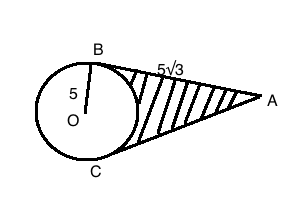a) $25 (\sqrt{3}-\frac{\pi}{3})$

b) $25 (\frac{\sqrt{3}}{2}-\frac{\pi}{4})$

c) $25 (\sqrt{3}-\frac{\pi}{4})$

d) $25 (\sqrt{3}-\frac{\pi}{6})$

Solution:

In the triangle BOA, tan $\theta = \frac{5\sqrt{3}}{5} = \sqrt{3}$
Therefore, $\theta = 60$ degrees.

The shaded area = Area of two triangles – area of section of circle.
As the radius is perpendicular to a tangent, triangle OAB is a right angled triangle, with right angle at B, with area=$1/2*5*5\sqrt{3}$.
Hence area of both triangles= $2*1/2*5*5\sqrt{3}=25 \sqrt{3}$.
The angle BOA=$tan^{-1} \frac{5 \sqrt{3}}{5} = 60^{\circ}$.

Hence, angle BOC = 2*BOA = 120°
Hence the area of the section = area of circle/3 = $\frac{\pi (5^2)}{3}$.
Hence area of shaded portion= $25 (\sqrt{3} -\frac{\pi}{3})$

Question 27: The number of real-valued solutions of the equation $2^{x}+2^{-x}=2-(x-2)^{2}$ is:

a) 1

b) 2

c) infinite

d) 0

Solution:

The graphs of $2^{x}+2^{-x} and 2-(x-2)^{2}$ never intersect. So, number of solutions=0.

Alternate method:

We notice that the minimum value of the term in the LHS will be greater than or equal to 2 {at x=0; LHS = 2}. However, the term in the RHS is less than or equal to 2 {at x=2; RHS = 2}. The values of x at which both the sides become 2 are distinct; hence, there are zero real-valued solutions to the above equation.

Question 28: The product of the distinct roots of $\mid x^2 – x – 6 \mid = x + 2$ is

a) −16

b) -4

c) -24

d) -8

Solution:

We have, $\mid x^2 – x – 6 \mid = x + 2$

=> |(x-3)(x+2)|=x+2

For x<-2, (3-x)(-x-2)=x+2

=> x-3=1   =>x=4 (Rejected as x<-2)

For -2$\le\$x<3, (3-x)(x+2)=x+2    =>x=2,-2

For x$\ge\$3, (x-3)(x+2)=x+2   =>x=4

Hence the product =4*-2*2=-16

Question 29: If the coefficient of $x^8$ in the expansion $(px^2+\frac{1}{qx})^{13}$ equals the coefficient of $x^{-8}$ in $(px-\frac{1}{qx^2})^{13}$, what is the relationship between p and q?

a) p+q=0

b) p=q

c) pq=-1

d) pq=1

Solution:

Let the term with coefficient $x^8$ in the expansion $(px^2+\frac{1}{qx})^{13}$ be the $a^{th}$ term.
So, 2*a-(13-a) = 8
or a=7
So, the coefficient of $x^8$ is $^{13} C_7 *p^7*q^{-6}$

Similarly, the term with coefficient $x^{-8}$ in the expansion $(px-\frac{1}{qx^2})^{13}$ is the sixth term.
So, the coefficient of $x^{-8}$ is -$^{13} C_6 * p^6*q^{-7}$

As, both of them are equal, pq = -1

Question 30: A cricket camp invited 500 cricket players who were batsmen, bowlers, and all-rounders(who can both bat and bowl). 60% of the players were batsman and 70% of them were bowlers. 50% of the pure batsmen (who can only bat), 60% of the pure bowlers (who can only bowl) and 20% of the all-rounders were left-handed. How many right-handed players were part of the cricket camp?

a) 275

b) 360

c) 225

d) 210

Solution:

Given, there are 60% batsmen and 70% bowlers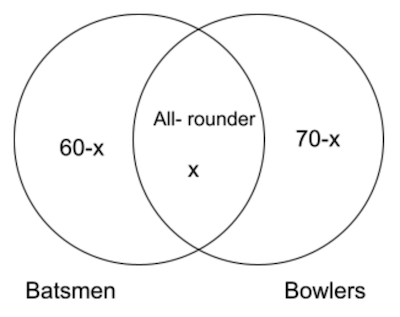So, (60-x)+x+(70-x)= 100

=> 130-x= 100

.’. x= 30%

So, the team has 30% of pure batsman, 30% of all rounders and 40% of pure bowlers.

Left handed players= (50% of 30% of the team)+(60% of the 40% of team)+(20% of 30% of the team)

= $\left(\frac{50}{100}\times\frac{30}{100}\times500\right)+\left(\frac{60}{100}\times\frac{40}{100}\times500\right)+\left(\frac{20}{100}\times\frac{30}{100}\times500\right)\ \ \ \$= 75+120+30= 225

The total number of right-handed players=500-225=275.

Question 31: A shopkeeper has 5 dozens of apples. On selling 12 apples for Rs.600, he incurs a loss equal to the cost price of 2 apples. At what total price should he mark the remaining 4 dozens so that even after giving 20% discount, he would have an overall gain of 10%?

Solution:

CP = SP + loss
So, CP of 12 apples = SP of 12 apples+ CP of 2 apples
=> CP of 10 apples = SP of 12 apples
SP of 12 apples = Rs.600
=> CP of 10 apples = Rs.600
CP of 1 apple = Rs. 60
Total CP = Rs. (5 * 12 * 60) = Rs. 3600
Required overall gain = 10%
Required total SP = Rs. 3600 + 10% of Rs. 3600 = Rs. 3960
Remaining apples = 4 dozens
Required SP for 4 dozens = Rs. (3960 – 600) = Rs.3360
According to the question,
MP – 20% of MP = Rs. 3360
Or, MP = Rs. 4200
Hence, 4200 is the correct answer.

Question 32: The number of positive integral solutions for the equation
$\log_{2}\frac{3x-7}{2x+3} \leq 0$

a) 10

b) 8

c) 16

d) Infinite

Solution:

We have $\log_{2}\frac{3x-7}{2x+3} \leq 0$
=> $\frac{3x – 7}{2x + 3}\leq 1$
=> 3x – 7 ≤ 2x + 3
=> x ≤ 10
=> We know that logarithm is defined only for positive integers, so
=> $\frac{3x – 7}{2x + 3}\geq 0$
=> x > 7/3 or x < -3/2 but we have been asked only about the positive solutions so we can ignore the negative x. Hence x > 7/3
So x can take all integer values between 7/3 and 10 (10 included).
Hence required values are 3,4,5,6,7,8,9 and 10

Question 33: Which of the following can’t be the sum of the squares of the roots of the equation: $x^2 + (p+6)x + (p+\dfrac{21}{4}) = 0$, if both the roots are imaginary.

a) $\dfrac{21}{4}$

b) $\dfrac{17}{4}$

c) $\dfrac{15}{4}$

d) $\dfrac{13}{4}$

Solution:

We are given that root are imaginary, therefore,

$\Rightarrow$ $(p+6)^2 – 4(p + \dfrac{21}{4}) < 0$

$\Rightarrow$ $p^2+12p+36-4p-21<0$

$\Rightarrow$ $p^2+8p+15<0$

$\Rightarrow$ $(p + 5)(p + 3)<0$

Therefore, p = (-5, -3) … (1)

Let ‘a’ and ‘b’ be the roots of the given quadratic equation,

$\Rightarrow$ $a^2+b^2 = (a+b)^2 – 2ab$
$\Rightarrow$ $a^2+b^2 = (p+6)^2 – 2(p + \dfrac{21}{4})$
$\Rightarrow$ $a^2+b^2 = p^2 + 10 p + \dfrac{51}{2}$
$\Rightarrow$ $a^2+b^2 = p^2 + 10 p + 25 – 25 + \dfrac{51}{2}$
$\Rightarrow$ $a^2+b^2 = (p+5)^2 + \dfrac{1}{2}$  … (2)

We know that  $- 5 < p < -3$

$\Rightarrow$  $- 5 + 5 < p+5 < -3 + 5$
$\Rightarrow$  $0 < p+5 < 2$
$\Rightarrow$  $0 < (p+5)^2 < 4$
$\Rightarrow$  $0 + \dfrac{1}{2} < (p+5)^2 + \dfrac{1}{2}< 4 + \dfrac{1}{2}$
$\Rightarrow$  $\dfrac{1}{2} < a^2+b^2 < \dfrac{9}{2}$

Hence we can say that the sum of the squares of the roots of the given equation can’t be $\dfrac{21}{4}$. Hence, option A is the correct answer.

Question 34: The average of a group of 11 distinct positive integers is 57. If the average of the smallest 6 integers is 23, what is the maximum possible value of the largest integer?

Solution:

Average of 11 integers = 57
Sum of 11 integers = 57 * 11 = 627
Average of the smallest 6 integers = 23
Sum of the smallest 6 integers = 23 * 6 = 138
Sum of the other 5 integers = 627 – 138 = 489
To maximise the largest integer, all other integers must be minimised.
So, the least 6 integers must be (20, 21, 22, 24, 25, 26)
Other four integers must be 27, 28, 29, 30
Thus, the largest integer can be 489 – (27 + 28 + 29 + 30) = 375
Hence, 375 is the correct answer.

Question 35: The number of distinct pairs of integers (m,n), satisfying $\mid1+mn\mid<\mid m+n\mid<5$ is:

Solution:

Let us break this up into 2 inequations [ Let us assume x as m and y as n ]

| 1 + mn | < | m + n |

| m + n | < 5

Looking at these expressions, we can clearly tell that the graphs will be symmetrical about the origin.

Let us try out with the first quadrant and extend the results to the other quadrants.

We will also consider the +X and +Y axes along with the quadrant.

So, the first inequality becomes,

1 + mn < m + n

1 + mn – m – n < 0

1 – m + mn – n < 0

(1-m) + n(m-1) < 0

(1-m)(1-n) < 0

(m – 1)(n – 1) < 0

Let us try to plot the graph.

If we consider only mn < 0, then we get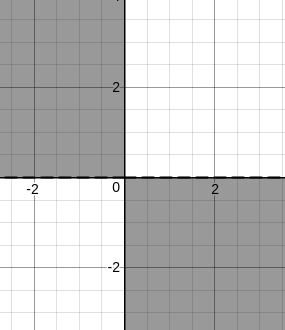But, we have (m – 1)(n – 1) < 0, so we need to shift the graphs by one unit towards positive x and positive y.

So, we have,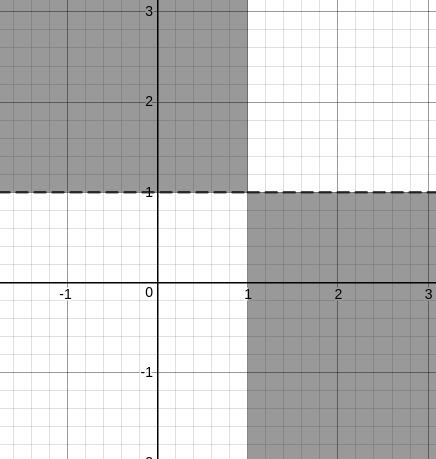But, we are only considering the first quadrant and the +X and +Y axes. Hence, if we extend, we get the following region.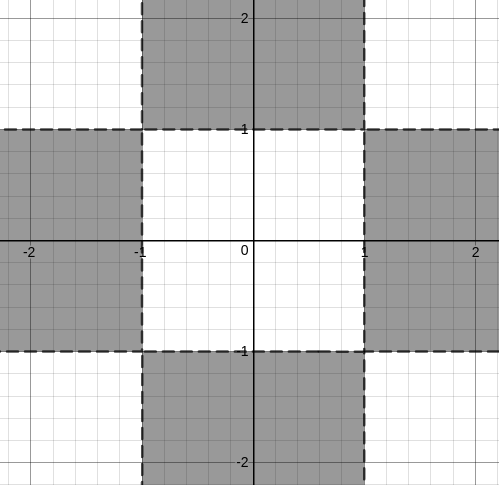So, if we look for only integer values, we get

(0,2), (0,3),…….

(0,-2), (0, -3),……

(2,0), (3,0), ……

(-2,0), (-3,0), …….

Now, let us consider the other inequation as well, in which |x + y| < 5

Since one of the values is always zero, the modulus of the other value is less than or equal to 4.

Hence, we get

(0,2), (0,3), (0,4)

(0,-2), (0, -3), (0, -4)

(2,0), (3,0), (4,0)

(-2,0), (-3,0), (-4,0)

Hence, a total of 12 values.

Question 36: A compound ‘M’ is to be created, one unit of which consists of 3 units of ‘X’, 4 units of ‘Y’ and 6 units of ‘Z’.  1 unit of X is made by A and B in the ratio 5:7 respectively, 1 unit of Y is made by B and C in the ratio 2:3 respectively, and 1 unit of Z is made by A and D in the ratio 1:4 respectively. What is the approximate percentage contribution of A in 1 unit of M?

a) 18.84%

b) 20.87%

c) 22.3%

d) 24.1%

Solution:

The ratio of A, B, C and D in X, Y and Z is given to be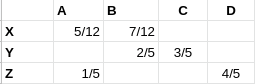Since 1 unit of M has 3X, 4Y and 6Z, we can show the contribution of A, B, C and D in 1 unit of M or 3 units of X, 4 units of Y and 6 units of Z.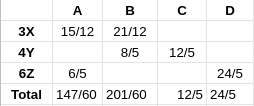Therefore 1 unit of M has $\frac{147}{60}$ units of A, $\frac{201}{60}$ units of B, $\frac{12}{5}$ units of C and $\frac{24}{5}$ units of D.

Also, the total quantity of 1 unit of M= (3+4+6) units= 13 units.

.’. Percentage contribution of A in 1 unit of M= $\frac{\frac{147}{60}}{13}\times100=49\times\frac{5}{13}\%=18.84\%\$

Question 37: Three lines divide a triangle in 5 areas. The areas of 3 parts have been shown in the figure and two unknown areas have been represented as x and y. Find the value of $\ \frac{\ x}{y}$ ?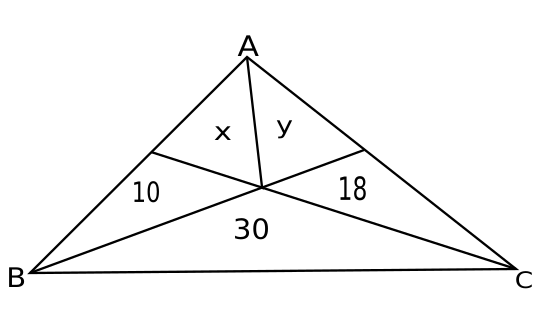a) $\ \frac{\ 6}{7}$

b) $\ \frac{\ 5}{6}$

c) $\ \frac{\ 4}{5}$

d) $\ \frac{\ 7}{8}$

Solution:

From figure,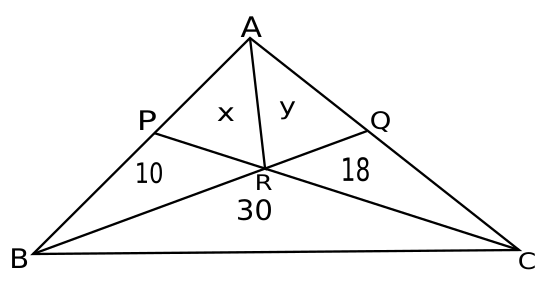In triangle ABC, BQ divides the triangle into two parts.

Since the altitude from B is same, the ratio of areas of triangles ABQ and CBQ will be the ratio of AQ and CQ.

=> $\ \frac{\ area\left(ABQ\right)}{area\left(CBQ\right)}$ = $\ \frac{\ AQ}{CQ}$

=> $\ \ \frac{\ 10+x+y}{48}=\ \frac{\ AQ}{CQ}$ …..(1)

Similarly RQ will divide the triangle ARC in the ratio of AQ and CQ.

=> $\ \ \frac{\ y}{18}=\ \frac{\ AQ}{CQ}$…….(2)

From 1 and 2, $\ \ \frac{\ y}{18}=\ \frac{\ 10+x+y}{48}$

=> 30y-18x=180…….(3)

Similarly, the equation for triangles ACP and BCP can be written as,

$\ \ \frac{\ x}{10}=\ \frac{\ 18+x+y}{40}$

=> 3x-y=18 …….(4)

From 3 and 4, x =10, y=12

Hence, $\ \frac{\ x}{y}$=$\ \frac{\ 5}{6}$

Question 38: How many disticnt positive integer-valued solutions exist to the equation $(x^{2}-7x+11)^{(x^{2}-13x+42)}=1$ ?

a) 8

b) 4

c) 2

d) 6

Solution:

$(x^{2}-7x+11)^{(x^{2}-13x+42)}=1$

if $(x^{2}-13x+42)$=0 or $(x^{2}-7x+11)$=1 or $(x^{2}-7x+11)$=-1 and $(x^{2}-13x+42)$ is even number

For x=6,7 the value $(x^{2}-13x+42)$=0

$(x^{2}-7x+11)$=1 for x=5,2.

$(x^{2}-7x+11)$=-1 for x=3,4 and for X=3 or 4, $(x^{2}-13x+42)$ is even number.

.’. {2,3,4,5,6,7} is the solution set of x.

.’. x can take six values.

Question 39: You travel by Delhi Metro every day from Botanical Garden, Noida to Hauz Khas, Delhi. At Hauz Khas metro station, you use an escalator to get out of the station. The escalator takes 80 seconds to get you down. One day, the escalator was not working and you walk up the escalator in 50 seconds. How many minutes does it approximately take you to walk up the working escalator?

a) 1.5 minutes

b) 2.2 minutes

c) 2.8 minutes

d) 2.6 minutes

Solution:

It is given that by using the escalator, one can get out of the station in 80 sec.

Let us consider the number of steps =80

Speed of the escalator = 1 step/sec

When the escalator was not working, the time taken by a person = 50 sec

The speed of the person = 80/50=8/5 steps/sec

Time taken by a person walking down a moving escalator = $\ \frac{\ 80}{\ \frac{\ 8}{5}-1}$

=$\ \frac{\ 80}{\ \frac{\ 3}{5}}$

=2.22 min

Question 40: 3 persons Akram, Bikram, Chandan take part in a race. Akram can give Bikram a head start of 64 feet. The head start that Chandan can give Bikram is 40 feet longer than the head start that he can give to Akram. If it is known that one person is twice as fast as one of the other two persons and the length of the track (in feet) is an integer, what is the length of the track (in feet)?

a) 128

b) 420

c) 320

d) More than one of the above

Solution:

Let the length of the race be $x$ m. Let us denote the speeds of Akram, Bikram, and Chandan with $a, b$ and $c$ respectively.
Akram can give Bikram a head start of 64 feet.

=> $\frac{x}{a} = \frac{x-64}{b}$
=> $\frac{a}{b} = \frac{x}{x-64}$ ————-(1)

The head start that Chandan can give Bikram is 40 feet longer than the head start that he can give to Akram.

Let us assume that Chandan gives Akram a head start of $y$ feet.
Now, we can form 2 equations.

$\frac{x}{c} = \frac{x-y}{a}$
=> $\frac{a}{c} = \frac{x-y}{x}$ ———-(2)

$\frac{x}{c} = \frac{x-y-40}{b}$
$\frac{b}{c} = \frac{x-y-40}{x}$ ————(3)

Dividing (2) by (3), we get,
$\frac{a}{b} = \frac{x-y}{x-y-40}$ ———————–(4)

Equating (1) and (4), we get,

$\frac{x}{x-64} = \frac{x-y}{x-y-40}$ ———-(5)

We know that the ratio of speeds of 2 of the 3 persons is 2:1.

If the value of (1) is 2, then $x$ will be equal to $128$.
Substituting the value of $x$ in (5), we get,

$2 = \frac{128-y}{88-y}$
$176-2y = 128-y$
$y = 48$

Let us assume the value of (2) to be $\frac{1}{2}$.

$\frac{1}{2} = \frac{x-y}{x}$
=> $y = \frac{x}{2}$
Substituting the value of $x$ in (5), we get,

$\dfrac{x}{x-64} = \dfrac{\frac{x}{2}}{\frac{x}{2}-40}$
$\dfrac{x}{x-64} = \frac{x}{x-80}$

As we can see, the equation is inconsistent and hence, we can eliminate this case.

Let us assume the value of (3) to be $\frac{1}{2}$.

$\frac{1}{2} = \frac{x-y-40}{x}$
=> $(y+40) = \frac{x}{2}$
$x = 2y+80$

Substituting this relationship in (5), we get,
$\frac{2y+80}{2y+16} = \frac{y+80}{y+40}$
$(2y+80)(y+40) = (2y+16)(y+80)$
$2y^2+160y+3200 = 2y^2+176y+1280$
$16y = 1920$
=> $y = 120$
=> $x = 320$ feet.

As we can see, the length of the track can be $128$ feet or $320$ feet. Therefore, option D is the right answer.

Question 41: In a certain infinite geometric progression, the sum of any three consecutive terms equals 26 times the sum of all the terms that follow them. What is the ratio of any term of the progression to the sum of all the terms that follow it?

a) 1

b) 1/2

c) 2

d) -1

Solution:

Let the geometric progression be $a,ar,ar^2,ar^3,….$
It is given that,
$a+ar+ar^2=26(\frac{ar^3}{1-r})$
$=>1-r^3=26r^3$
$=>27r^3=1$
$=>r=\frac{1}{3}$

The required ratio is,
$\frac{a}{\frac{ar}{1-r}}=\frac{1-r}{r}=2$

Question 42: If $x=(4096)^{7+4\sqrt{3}}$, then which of the following equals to 64?

a) $\frac{x^{7}}{x^{2\sqrt{3}}}$

b) $\frac{x^{\frac{7}{2}}}{x^{\frac{4}{\sqrt{3}}}}$

c) $\frac{x^{\frac{7}{2}}}{x^{2{\sqrt{3}}}}$

d) $\frac{x^{7}}{x^{4\sqrt{3}}}$

Solution:

$x=2^{12\left(7+4\sqrt{\ 3}\right)}$.

$x^{\frac{7}{2}}=2^{42\left(7+4\sqrt{\ 3}\right)}$

$x^{2\sqrt{\ 3}}=2^{24\sqrt{\ 3}\left(7+4\sqrt{\ 3}\right)}$

$\frac{x^{\frac{7}{2}}}{x^{2{\sqrt{3}}}}$ = $2^{\left(7+4\sqrt{\ 3}\right)\left(42-24\sqrt{\ 3}\right)}=2^{\left(7+4\sqrt{\ 3}\right)\left(7-4\sqrt{\ 3}\right)6}$ =$2^6$.

Question 43: A man standing on the line joining the two poles finds that the top of the poles make an angle of elevation of $60^\circ$ and $45^\circ$ respectively. After walking for sometime towards the other pole, the angles change to $30^\circ$ and $60^\circ$ respectively. The ratio of the height of the poles is :

a) $\frac{\sqrt{3}-1}{2}$

b) $\frac{\sqrt{3}+1}{3}$

c) $\frac{\sqrt{3}-1}{4}$

d) $\frac{\sqrt{3}+1}{4}$

Solution:

Let ‘a’ and ‘b’ be the heights of the two poles

X be the initial position of the man and the angles of elevation be $60^0$ and $45^0$

Distance between pole 1 and X  = $\ \frac{\ a}{\sqrt{\ 3}}$ and distance between pole 2 and X = b

Let Y be the position of the man after walking sometime towards the other pole =

Distance between Y and pole 1 = $\ \ a\sqrt{\ 3}$ and distance between the pole 2 and Y = $\ \frac{\ b}{\sqrt{\ 3}}$

Since the distance between the poles will remain the same

$\ \frac{\ a}{\sqrt{\ 3}}$ + b = $\ \ a\sqrt{\ 3}$ + $\ \frac{\ b}{\sqrt{\ 3}}$

$\ \frac{\ a}{b}=\ \ \frac{\ \sqrt{\ 3}-1}{2}$

Question 44: An alloy is prepared by mixing three metals A, B and C in the proportion 3 : 4 : 7 by volume. Weights of the same volume of the metals A. B and C are in the ratio 5 : 2 : 6. In 130 kg of the alloy, the weight, in kg. of the metal C is

a) 48

b) 84

c) 70

d) 96

Solution:

Let the volume of Metals A,B,C we 3x, 4x, 7x

Ratio weights of given volume be 5y,2y,6y

.’. 15xy+8xy+42xy=130 => 65xy=130 => xy=2.

.’.`The weight, in kg. of the metal C is 42xy=84.

Question 45: One spherical ball is placed over a hollow cylindrical box. The radius of the sphere is 14 cm, while the cylinder is of radius 7 cm. If the height of the cylinder is 10 cm, what is the total height of the figure?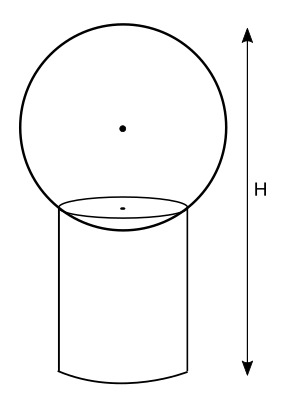a) 24+7$\sqrt{\ 3}$ cm

b) 24 cm

c) 14+7$\sqrt{\ 3}$ cm

d) 24+14$\sqrt{\ 3}$ cm

Solution:

The figure can be represented as :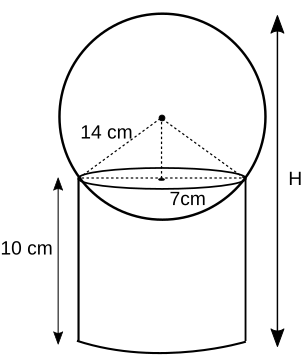The triangle formed is an equilateral triangle with the side of length 14cm

The height of an equilateral triangle= $\frac{\sqrt{\ 3}}{2}\cdot a$

=$\frac{\sqrt{\ 3}}{2}\cdot 14$

= $7\sqrt{\ 3}$

Height = 10+ $7\sqrt{\ 3}$+ 14 cm

= 24+$7\sqrt{\ 3}$

Option A is the correct answer.

Question 46: If   log m=300$\times$log1.0033333..
What is the approximate value of m?

a) 2.5

b) 2.6

c) 2.7

d) 3

Solution:

log m = 300 log(1.003333)

log m =300 log(1+$\frac{1}{300}$)

log m =$(1+\frac{1}{300})^{300}$

m =(1+$\frac{1}{300})^{300}$

Binomial Expansion of $(1+x)^{n}$=1+$^{n}C_1(1)x^{n-1}+^{n}C_2(1)^{2}x^{n-2}$……………..

$(1+\frac{1}{300})^{300}$=1+$^{300}C_1(1)^{299}\frac{1}{300}+^{300}C_2(1)^{298}\frac{1}{300}^{2}+^{300}C_3(1)^{297}\frac{1}{300}^{3}$……………}

$(1+\frac{1}{300})^{300}$=1+$300 * \frac{1}{300}+\frac {300*299}{2}*(\frac{1}{300})^{2}+\frac{300*299*298}{1*2*3}*(\frac{1}{300})^{3}+ \frac{300*299*298*297}{1*2*3*4}*(\frac{1}{300})^{4}$……………}

=1+1+0.498+0.165+ 0.041….

(Since $\frac{1}{300}^{4}$ and the succeeding terms are very small, they can be ignored because the fraction at the denominator is increasing and at the numerator is decreasing with the subsequent terms of bionomial.

Further if we observe the fourth term of the binomial expansion:

= $\frac{300*299*298*297}{1*2*3*4}*(\frac{1}{300})^{4}$

= $\frac{ 300}{300} *\frac{ 299}{300}*\frac{ 298}{300}*\frac{ 297}{300}*\frac{ 1}{4!}$

we can observe that factors $\frac{300}{300}$ , $\frac{ 299}{300}$ ,$\frac{ 298}{300}$ ,$\frac{ 297}{300} \approx$ 1 and with every subsequent term $\frac{1}{n!}$ will get smaller. Thus we can neglect those terms of the expansion. )

=1+1+0.498+0.165+ 0.041….

= {2.704+….}

This can be approximated to 2.7

Hence, C is the correct answer.

In general, when $n$ is very large, the value of $(1+\frac{1}{n})^n$ tends to the constant ‘e’ which is approximately equal to 2.718

Question 47: A region is in-plane x and y such that $x^2+y^2\le\ 25$and  |y| $\ge\ \frac{\ 5}{\sqrt{\ 2}}$. Find the area of the region.

a) $25\left(\ \frac{\ \pi\ }{2}+2\right)$

b) $25\left(\ \frac{\ \pi\ }{2}-2\right)$

c) $25\left(\ \frac{\ \pi\ }{2}-1\right)$

d) $25\left(\ \frac{\ \pi\ }{2}+1\right)$

Solution:

We have,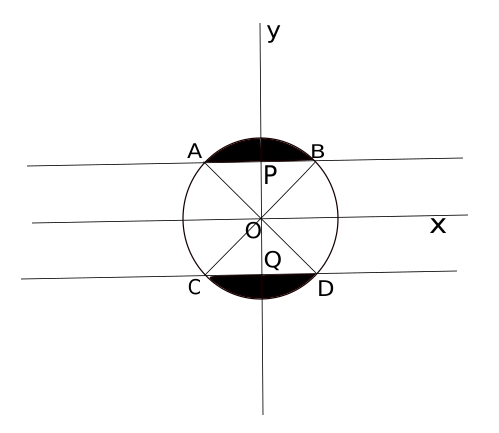Now, since AO = BO = 5 ……(1)
OP = $\ \frac{\ 5}{\sqrt{2}}$
In right triangle OPB, PB = $\sqrt{{\ 5^2-\left(\frac{5}{\sqrt{\ 2}}\right)}^2}$ = $\ \frac{\ 5}{\sqrt{2}}$
AB = $2\times\ PB\ =\ 2\times\ \ \frac{\ 5}{\sqrt{\ 2}}$ =$5\sqrt{2}$ …..(2)

Hence AOB is a right triangle.  (from 1 and 2)

The area of shaded region = 2(Area of quarter circle- Area of the triangle AOB) = 2($\ \frac{\ \pi\ }{4}\times\ 25\ -\ \ \ \ \frac{\ 1}{2}\times\ 5\times\ 5$)

= $25\left(\ \frac{\ \pi\ }{2}-1\right)$

Question 48: If $x=8-\sqrt{32}$ and $y=2+\sqrt{2}$, then $\left(x+\frac{1}{y}\right)^2$ is given by:

a) $\frac{16}{25}x^2$

b) $\frac{64}{81}y^2$

c) $\frac{25}{16}y^2$

d) $\frac{81}{64}x^2$

Solution:

$x=8-\sqrt{32}$ and $y=2+\sqrt{2}$,

We have to find the value of $\left(x+\frac{1}{y}\right)^2$

$\left(8-\sqrt{\ 32}+\ \frac{\ 1}{2+\sqrt{\ 2}}\right)^2$

$(\ \frac{\ 8\left(2+\sqrt{\ 2}\right)-\sqrt{\ 32}\left(2+\sqrt{\ 2}\right)+\ \ 1}{2+\sqrt{\ 2}})^2$

$\left(\ \frac{\ 9}{2+\sqrt{\ 2}}\right)^2$

$\ \frac{\ 81}{6+2\sqrt{\ 2}}$

$\left(\ \frac{\ 1}{y}\right)^2=\ \left(\frac{\ 1}{2+\sqrt{\ 2}}\right)^2$ = $6+2\sqrt{\ 2}$

=$\left(\ \frac{\ 2-\sqrt{\ 2}}{2}\right)^{^2}$

=$\ \frac{\ 6-4\sqrt{2\ }}{4}$

=$\ \frac{\ 3-2\sqrt{2\ }}{2}$

$x^2=64+32-64\sqrt{\ 2}$

=$96-64\sqrt{\ 2}$

=32($3-2\sqrt{\ 2}$) = 32*$2y^2$

we get, $x^{2\ }=\ 64y^2$

$\ \frac{\ 81}{y^2}=\ \frac{\ 81}{64}x^2$

Alternative solution,

$xy=\left(8-\sqrt{\ 32}\right)\left(2+\sqrt{\ 2}\right)=4\sqrt{\ 2}\left(\sqrt{\ 2}-1\right)\times\ \sqrt{\ 2}\left(\sqrt{\ 2}+1\right)=8\left(2-1\right)=8$

(As $xy=8\ \longrightarrow\ y=\dfrac{x}{8}$)

$\left(x+\frac{1}{y}\right)^2=\left(\frac{\left(xy+1\right)}{y}\right)^2=\left(\frac{\left(xy+1\right)\times\ x}{8}\right)^2=\left(\frac{\left(8+1\right)\times\ x}{8}\right)^2=\frac{81}{64}\times\ x^2$

Question 49: In how many ways can a pair of integers (x , a) be chosen such that $x^{2}-2\mid x\mid+\mid a-2\mid=0$ ?

a) 6

b) 5

c) 4

d) 7

Solution:

$x^{2}-2\mid x\mid+\mid a-2\mid=0$

where x>= 0 and x>=2

$x^2-2x+a-2\ =0$ Using quadratic equation we have $x=\ 1+\sqrt{\ 3-a}\ and\ x=1-\sqrt{\ 3-a}$ Only two integer values are possible

a=2 and a=3. So corresponding “x” values are x=1 and a=3, x=2 and a=2, x=0 and a=2

where x>=0 and x<2

Applying the above process we get x=1 and a=1

where x<0 and x>=2 we get a=3 and x=-1 , a=2 and x=-2

where x<0 and x<2 we get a=1 and x=-1

Hence there are total 7 values possible

Question 50: On a rectangular metal sheet of area 135 sq in, a circle is painted such that the circle touches two opposite sides. If the area of the sheet left unpainted is two-thirds of the painted area then the perimeter of the rectangle in inches is

a) $3\sqrt{\pi}(5+\frac{12}{\pi})$

b) $4\sqrt{\pi}(3+\frac{9}{\pi})$

c) $3\sqrt{\pi}(\frac{5}{2}+\frac{6}{\pi})$

d) $5\sqrt{\pi}(3+\frac{9}{\pi})$

Solution:

Let ABCD be the rectangle with length 2l and breadth 2b respectively.

Area of the circle i.e. area of painted region = $\pi\ b^2$.

Given, 4lb-$\pi\ b^2$=(2/3)$\pi\ b^2$.

=> 4lb=(5/3)$\pi\ b^2$.

=>l=$\frac{5\pi}{12}b$.

Given, 4lb=135 => 4*$\frac{5\pi}{12}b^2$=135 => b= $\frac{9}{\sqrt{\ \pi\ }}$

=> l=$\frac{15}{4}\sqrt{\ \pi\ }$
Perimeter of rectangle =4(l+b)=4($\frac{15}{4}\sqrt{\ \pi\ }$+$\frac{9}{\sqrt{\ \pi\ }}$ )=$3\sqrt{\pi}(5+\frac{12}{\pi})$.

Hence option A is correct.